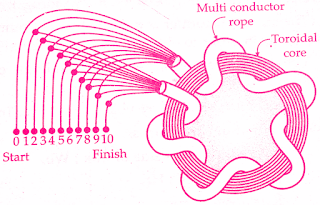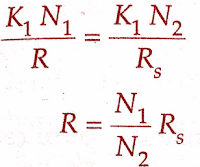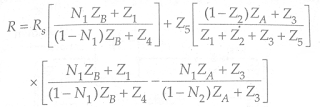# Transformer Ratio Bridges Working Principle

0
129

## What are Transformer Ratio Bridges:

### Transformer Ratio Bridges Working Principle:

#### E1 = E. N1/N  and E2 = E. N2/N#### (adsbygoogle = window.adsbygoogle || []).push({}); The magnetizing current is reduced by using a Toroidal Core.The added advantage of a toroidal core is that winding put on it has minimum leakage reactance giving an almost perfect coupling.The leakage reactance can be reduced further by using a special type of construction for the windings as shown in the below figure of transformer ratio bridges.This winding takes the form of a Multiconductor Rope.In order to obtain a decade of voltage division, the multiconductor rope has ten wires with successive sets of turns connected in series and a tapping is taken from each joint.#### The resistance of the windings can be reduced by using copper wire of heavy cross-section.A 4-decade ratio transformer is shown.The successive decades are obtained by using an arrangement similar to that in a Kelvin Varley slide.This transformer arrangement gives a ratio error of less than 1 part in 10⁴.### Measurement of Resistance By Transformer Ratio Bridges:

#### The circuit used for measurement of an unknown resistance, R, in comparison with a standard resistance, Rs is shown in the below figure.The position of the wiper is adjusted till the detector D shows null.(The detectors used are the same as for conventional ac bridges).Measurement of Resistance By Transformer Ratio Bridges#### Under balance conditions, the current through the detector is zero i.e., I1 = 12.#### A circuit used for measurement of low resistance is shown in the below figure.This circuit is similar to that of Kelvin’s double bridge.#### By using two transformers a form of Kelvin’s double bridge for measurement of low resistances may be devised as shown in the below figure, where#### If the impedances of leads i.e., Z1, Z2, Z3, Z4, and Z5 are small and the resistances R and Rs are of the same order, the unknown resistance is given by: R = (N1/N2)RsThis form of Kelvin’s bridge can, of course, only be used on ac but by plotting the values R against frequency, an extrapolation may be used to find the dc value of R with an accuracy of a few parts in a million.

READ HERE  Magnetostrictive Sensor Working Principle & Applications